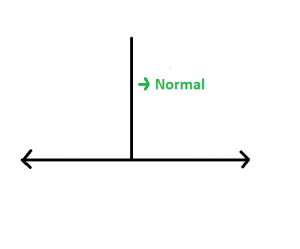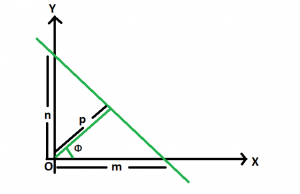# Length of the normal from origin on a straight line whose intercepts are given

Given the intercepts of a straight line on both the axes as m and n. The task is to find the length of the normal on this straight line from the origin.

Examples:

Input: m = 5, n = 3
Output: 2.57248

Input: m = 13, n = 9
Output: 7.39973

## Recommended: Please try your approach on {IDE} first, before moving on to the solution.

Approach: A normal to a line is a line segment drawn from a point perpendicular to the given line.Let p be the length of the normal drawn from the origin to a line, which subtends an angle Θ with the positive direction of x-axis as follows.Then, we have cos Θ = p / m and sin Θ = p / n
Since, sin2 Θ + cos2 Θ = 1
So, (p / m)2 + (p / n)2 = 1
We get, p = m * n / √(m2 + n2)

Below is the implementation of the above approach:

## C++

 `// C++ implementation of the approach ` `#include ` `using` `namespace` `std; ` ` `  `// Function to find the normal ` `// of the straight line ` `float` `normal(``float` `m, ``float` `n) ` `{ ` `    ``// Length of the normal ` `    ``float` `N = (fabsf(m) * fabsf(n)) ` `              ``/ ``sqrt``((fabsf(m) * fabsf(m)) ` `                     ``+ (fabsf(n) * fabsf(n))); ` ` `  `    ``return` `N; ` `} ` ` `  `// Driver code ` `int` `main() ` `{ ` `    ``float` `m = -5, n = 3; ` `    ``cout << normal(m, n); ` ` `  `    ``return` `0; ` `} `

## Java

 `// Java implementation of the approach ` `class` `GFG ` `{ ` ` `  `// Function to find the normal ` `// of the straight line ` `static` `float` `normal(``float` `m, ``float` `n) ` `{ ` `    ``// Length of the normal ` `    ``float` `N = (``float``) ((Math.abs(m) * Math.abs(n)) ` `            ``/ Math.sqrt((Math.abs(m) * Math.abs(m)) ` `                    ``+ (Math.abs(n) * Math.abs(n)))); ` ` `  `    ``return` `N; ` `} ` ` `  `// Driver code ` `public` `static` `void` `main(String[] args) ` `{ ` `    ``float` `m = -``5``, n = ``3``; ` `    ``System.out.println(normal(m, n)); ` `} ` `}  ` ` `  `// This code has been contributed by 29AjayKumar `

## Python3

 `# Python3 implementation of the approach ` `import` `math; ` ` `  `# Function to find the normal ` `# of the straight line ` `def` `normal(m, n): ` ` `  `    ``# Length of the normal ` `    ``N ``=` `((``abs``(m) ``*` `abs``(n)) ``/` `        ``math.sqrt((``abs``(m) ``*` `abs``(m)) ``+` `                  ``(``abs``(n) ``*` `abs``(n)))); ` ` `  `    ``return` `N; ` ` `  `# Driver code ` `m ``=` `-``5``; n ``=` `3``; ` `print``(normal(m, n)); ` ` `  `# This code is contributed ` `# by Akanksha Rai `

## C#

 `// C# implementation of the approach ` `using` `System; ` ` `  `class` `GFG ` `{ ` ` `  `// Function to find the normal ` `// of the straight line ` `static` `float` `normal(``float` `m, ``float` `n) ` `{ ` `    ``// Length of the normal ` `    ``float` `N = (``float``)((Math.Abs(m) * Math.Abs(n)) /  ` `                       ``Math.Sqrt((Math.Abs(m) * Math.Abs(m)) +  ` `                                 ``(Math.Abs(n) * Math.Abs(n)))); ` ` `  `    ``return` `N; ` `} ` ` `  `// Driver code ` `public` `static` `void` `Main() ` `{ ` `    ``float` `m = -5, n = 3; ` `    ``Console.Write(normal(m, n)); ` `} ` `}  ` ` `  `// This code is contributed by Akanksha Rai `

## PHP

 ` `

Output:

```2.57248
```

Attention reader! Don’t stop learning now. Get hold of all the important DSA concepts with the DSA Self Paced Course at a student-friendly price and become industry ready.

My Personal Notes arrow_drop_upProgram Analyst Trainee,Cognizant

If you like GeeksforGeeks and would like to contribute, you can also write an article using contribute.geeksforgeeks.org or mail your article to contribute@geeksforgeeks.org. See your article appearing on the GeeksforGeeks main page and help other Geeks.

Please Improve this article if you find anything incorrect by clicking on the "Improve Article" button below.